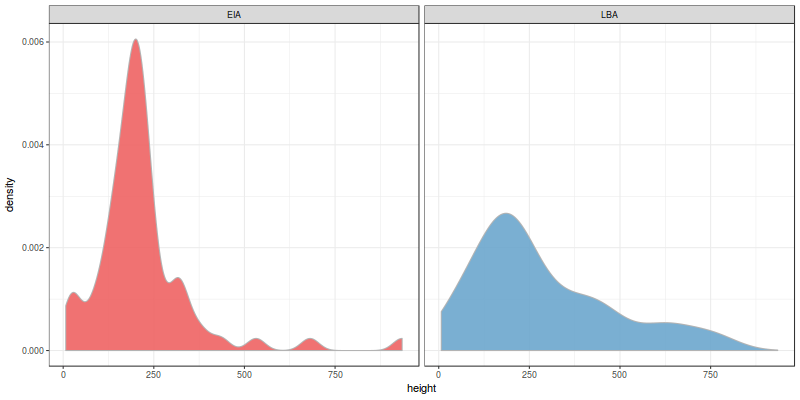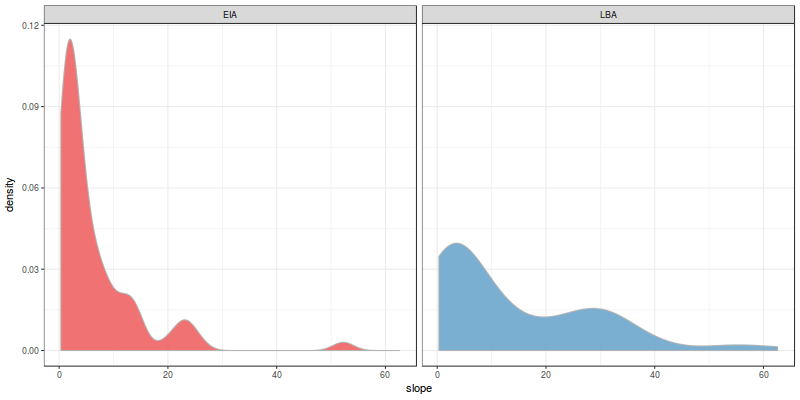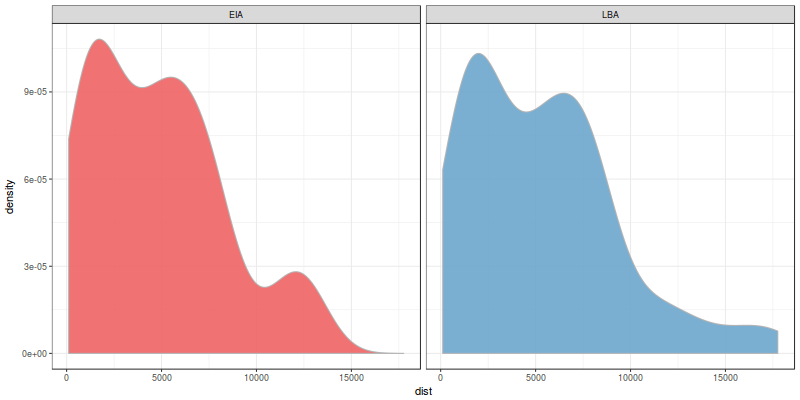[This article was first published on R – The Past by Numbers, and kindly contributed to R-bloggers]. (You can report issue about the content on this page here)
Want to share your content on R-bloggers? click here if you have a blog, or here if you don't.

Almost all statistical tests that archaeologists and historians use are based on the concept of Null Hypothesis Significance Testing or NHST. If someone told you that you should be using some stats in this paper this is probably what they mean for it. The comment is usually accompanied by a reference to a thick statistics manual where you repeatedly find a concept called p-value and other obscure terms.

A good understanding of p-values and NHST is essential for the current scientist; otherwise you won’t understand the results of a large percentage of papers you should be reading. The approach itself is rather simple, but current teaching of statistics bury it into a myriad of complex tests that you are supposed to learn in an almost ritualistic way, thus making everything more complicated than it really is (T-test, Chi-square test, Kruskal-Wallis test…seriously, who chooses these names??).

Let’s take a step back and see what NHST is really about.

# the concept of the null hypothesis

Imagine that you manage a lab and you need to test if a newly developed antibiotic drug kills bacteria. The trial seems straightforward: you put some of the new drug into a Petri dish with the bacteria and you wait to see if it works (also, apologies for my ignorance on lab trials to any lab technician that could be listening…). Your working hypothesis is that the drug is effective and kills bacteria, so what are the possible outcomes?

1. it works! bacteria are dead and the new drug is effective
2. it doesn’t work and bacteria are still alive.

In the first case we would accept the working hypothesis while in the second one we would reject it. However, it is not as simple as it seems because you could have lots of grey results between the 2 extremes: some bacteria may still be alive, bacteria could be dying for different causes than the drug, or even by pure chance.

Someone came up with a simpler approach to this problem that can be summed up as follows: what are the chances that you get the same result by pure chance? If these chances (known as the p-value of the test) are low enough then we could reject the idea of pure chance, thus confirming that in the trial something was killing bacteria. This is NHST: if we can reject the null hypothesis of pure chance with a good degree of significance then the alternate hypothesis of something going on is selected as a better explanation of the evidence.

This degree of significance is in reality defined as a threshold for the p-value; if the chances are typically lower than 5% (p-value < 0.05) then we will reject the null hypothesis.

# when to apply and when not to apply NHST

This is the type of scenarios where NHST was originally developed because in a controlled environment you can only design experiments with 2 results (it works or it doesn't work). However, this binary outcome is not necessarily found in other disciplines and specially it is problematic in archaeology.

Imagine that you are studying settlement patterns in late prehistoric Europe and you want to identify the geographical properties of these settlements. You would test if the gradient of terrain or slope is relevant here by testing the null hypothesis that it is not relevant.

Would you?

Really? If you think about it this null hypothesis is meaningless because we know that you won't be living at a slope of 40 degrees and hanging from a rope. You will obviously reject the null hypothesis! Moreover, this result doesn't mean that slope was particularly relevant during late prehistory because slope is always relevant to human settlements for obvious functional reasons: we don't have wings to fly.

The choice of a meaningless null hypothesis is a classic example of bad statistics; you should always evaluate if the approach you are applying makes sense or you need to tackle the research question with a different method.

What you could certainly do is to assess if the geographical features exhibited by settlements changed over time. Let's see how NHST works in practice.

# late prehistory in the NE Iberian Peninsula

The transition from Late Bronze Age (LBA) to Early Iron Age (EIA) in current Catalonia has been extensively studied during the last decades because of its interesting dynamics. Population seemed to shift from high-elevation pastures towards low-level agricultural regions; at the same time you find an increasing amount of artefacts brought to this area though long-range trade. Both processes seemed to increase the level of regional connectivity so…can we test this?

We will use here a dataset collected by Maria Yubero-Gómez who studied the period for her PhD and was published in:
* Yubero-Gómez, M., Rubio-Campillo, X., López-Cachero, F.J. and Esteve-Gràcia, X., 2015. Mapping changes in late prehistoric landscapes: a case study in the Northeastern Iberian Peninsula. Journal of Anthropological Archaeology, 40, pp.123-134. https://doi.org/10.1016/j.jaa.2015.07.002

To load the simplified dataset into R:

```sites <- read.csv("https://git.io/v9XNs")
str(sites)
```

You have here 5 variables:

• the name of the archaeological site
• distance to the closest natural route (i.e. main routes you would take to cross the region)
• slope of the site in degrees
• height in meters
• period of the site (LBA or EIA; if a site was active during both periods it will have 2 entries)## perform Exploratory Data Analysis

Let’s first focus on height. The first thing you need to do in any quantitative analysis is to explore the dataset with some plots:

```library(ggplot2)
ggplot(sites, aes(x=height)) + geom_density(col="grey70", aes(fill=period, group=period), alpha=0.9) + facet_wrap(~period) + scale_fill_manual(values=c("indianred2", "skyblue3")) + theme_bw() + theme(legend.position="none")
```Empirical density estimates of height (m.) per period

These are the density estimates for the evidence we collected. The distribution looks different for both periods, don’t they? Let’s take a look at the other 2 variables (slope and distance to natural routes):

```ggplot(sites, aes(x=slope)) + geom_density(col="grey70", aes(fill=period, group=period), alpha=0.9) + facet_wrap(~period) + scale_fill_manual(values=c("indianred2", "skyblue3")) + theme_bw() + theme(legend.position="none")
```Empirical density estimates of slope (degrees) per period

and the last one:

```ggplot(sites, aes(x=dist)) + geom_density(col="grey70", aes(fill=period, group=period), alpha=0.9) + facet_wrap(~period) + scale_fill_manual(values=c("indianred2", "skyblue3")) + theme_bw() + theme(legend.position="none")
```Empirical density estimates of distance to natural route (m,) per period

They don’t look as different as the slope. Specifically, the distribution of distances seems almost identical! However, the human eye is too good at identifying patterns so we may be finding similarities where there are not; we need to statistically test if they are different.

## test the null hypothesis

Our null hypothesis can be defined as follows: the distribution of slopes is the same for both periods. If we cannot reject this null hypothesis then it means that the differences we see in the plots are caused by pure chance; on the other hand, if we can reject it then we can say that there was a change on the relevance of slope over the transition LBA-EIA.

The perfect test to evaluate this null hypothesis is known as Kolmogorov-Smirnov test. The input of the test are the 2 distributions and the output is the probability that they were created by the same process. To summarize:

• If p-value > 0.05 then it means that there was a change between the 2 periods
• If p-value < 0.05 then it means that there was no significant change from LBA to EIA.

Let's create 2 new data frames (one for each period) and apply the Kolmogorov-Smirnov test to the 3 variables:

```lbaSites <- subset(sites, period=="LBA")
eiaSites <- subset(sites, period=="EIA")

ks.test(eiaSites\$slope, lbaSites\$slope)
ks.test(eiaSites\$height, lbaSites\$height)
ks.test(eiaSites\$dist, lbaSites\$dist)
```

The results for slope should be something like:

```Two-sample Kolmogorov-Smirnov test

data:  eiaSites\$slope and lbaSites\$slope
D = 0.27647, p-value = 0.006207
alternative hypothesis: two-sided
```

Ignore the warning as it is not relevant right now. The p-value you see is extremely low so we can safely reject the null hypothesis that the distribution of slope does not vary over the transition. Nice! The same can be said for elevation as the value is also low; however, the p-value of distances is really, really (really) high. The chances are over 90% so we cannot reject in this case the null hypothesis that the typical distance to natural route changed from LBA to EIA.

## final remarks

Some last comments on this analysis:

• Attention! A low p-value does not mean that the difference between distributions is large. You can have a very significant yet small difference between distributions and it would give you a very low p-value (e.g. all sites at an elevation of 200m against all sites at an elevation of 220m.).
• The choice of 0.05 as the significance threshold is a convention. Yes, we could choose 0.041 or 0.1 but you will see 0.05 as the limit in 90% of papers (also, if you see a paper using 0.1…don't trust the results…seriously)
• there are like 2023 statistical tests besides K-S for any type of variable: categorical, discrete, multivariate…in any case I think KS is one of the most useful for archaeologists

The approaches based on Null-Hypothesis Significance Testing have a lot of issues and there is a current debate on science about the appropriateness of the method. Alternatives such as Bayesian inference and model selection are becoming increasingly popular. However, NHST is still the standard statistical approach in archaeology so I hope this post was useful to understand the philosophy behind the method.